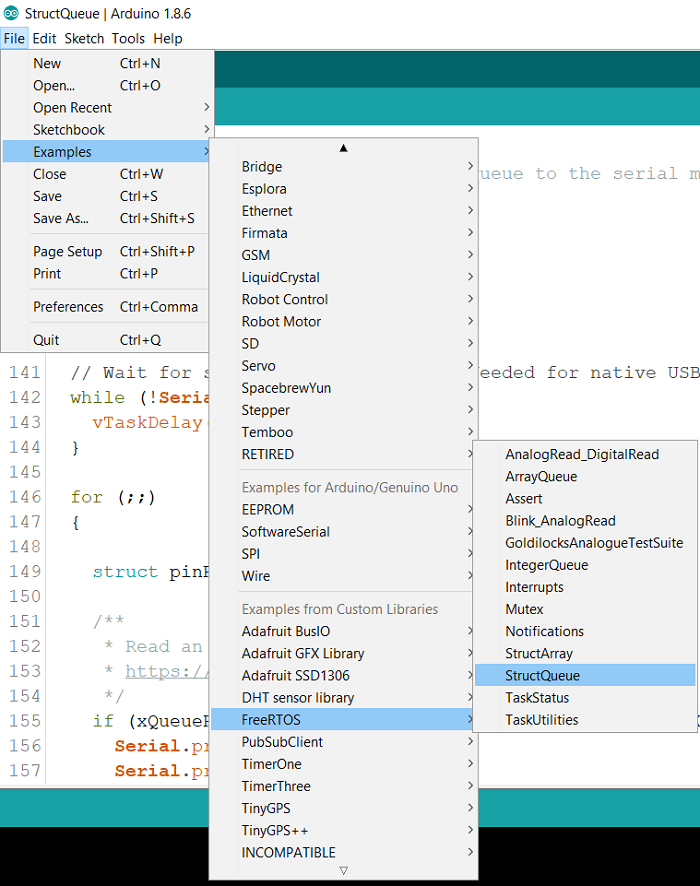# Queue in FreeRTOS in Arduino

ArduinoHardwareSoftware & Coding

Queue is a data structure that helps exchange data between different tasks or between tasks and interrupts. It holds a finite number of items (defined at the time of initialization) and operates in the FIFO mode.

We will walk through an example that comes in with the FreeRTOS library, to understand queues.

You can find the example in − File → Examples → FreeRTOS → StructQueue.In this code, two tasks read analog values from different analog pins, and pass these values in a queue. Another task reads the values from the queue and prints them onto the Serial Monitor. There is a fourth blink task which keeps working in parallel, and has nothing to do with the queue.

We begin with the inclusion of the libraries −

// Include Arduino FreeRTOS library
#include <Arduino_FreeRTOS.h>

// Include queue support
#include <queue.h>

Next, we define a struct containing two integers, one for the pin number and other for the pin value.

// Define a struct
int pin;
int value;
};

Then, a QueueHandle_t object is defined, which will then be used within Setup.

/*
* Declaring a global variable of type QueueHandle_t
*
*/
QueueHandle_t arrayQueue;

Within Setup, we create the queue and give it a size of 10 pinRead structs (it can store 10 such structs at a time). If the queue is created properly, then we create the 4 tasks. The Serial task is given the highest priority (2), the two analogRead tasks are given lower priority (1) and the blink task is given the lowest priority (0). Nothing happens in the loop.

void setup() {
/**
* Create a queue.
* https://www.freertos.org/a00116.html
*/
structQueue = xQueueCreate(10, // Queue length
sizeof(struct pinRead) // Queue item size
);
if (structQueue != NULL) {
// Create task that consumes the queue if it was created.
"Serial",     // A name just for humans
128,          // This stack size can be checked &
NULL,
2,            // Priority, with 3 (configMAX_PRIORITIES -
1) being the highest, and 0 being the
lowest.
NULL);
// Create task that publish data in the queue if it was created.
128,                  // Stack size
NULL,
1,                    // Priority
NULL);
// Create other task that publish data in the queue if it was created.
128,               // Stack size
NULL,
1,                 // Priority
NULL);
}
128,        // Stack size
NULL,
0,          // Priority
NULL );
}
void loop() {}

The definition of the two analogRead tasks follows next. Both are very similar to each other. One reads pin A0, and the other reads pin A1. Each task populates a pinRead struct and adds it to the queue. The third argument of xQueueSend is the maximum amount of time one should wait for space to become available in the Queue. By setting it to portMAX_DELAY, we make the task wait indefinitely for space to become available in the queue. See https://www.freertos.org/a00117.html

/**
*/
{
(void) pvParameters;

for (;;)
{
// Read the input on analog pin 0:

/**
* Post an item on a queue.
* https://www.freertos.org/a00117.html
*/

// One tick delay (15ms) in between reads for stability
}
}

/**
*/
{
(void) pvParameters;

for (;;)
{
// Read the input on analog pin 1:

/**
* Post an item on a queue.
* https://www.freertos.org/a00117.html
*/

// One tick delay (15ms) in between reads for stability
}
}

The Serial Task initializes Serial, and then whenever there is an item in the queue, it reads it into a local struct, and then prints the pin number and value on the Serial Monitor.

void TaskSerial(void * pvParameters) {
(void) pvParameters;

// Init Arduino serial
Serial.begin(9600);

// Wait for serial port to connect. Needed for native USB, on
LEONARDO, MICRO, YUN, and other 32u4 based boards.
while (!Serial) {
}

for (;;)
{
/**
* Read an item from a queue.
* https://www.freertos.org/a00118.html
*/
Serial.print("Pin: ");
Serial.print(" Value: ");
}
}
}

The blink task runs in parallel, without making any use of the Queue.

/*
*/
}